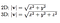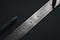# Introduction to Vector Norms

Vector calculations are important either directly or as a method that makes slight modifications to the learning algorithm such that the model generalizes better.

Vectors are used throughout the field of Machine Learning while formulating algorithms and processes to achieve the optimal target variable (y) after the training of the algorithm.

# What is vector norm?

Vector matrix operations often require you to calculate the length (or size) of a vector. In two dimensional space, the length of a vector is defined as,

The square root of the sum of the squares of the horizontal and vertical components.Length of vector for 2D and 3D space.

The length of a vector is what is referred to as the vector magnitude or vector norm.

The length of a vector is always non-negative. For non-zero vectors, the magnitude is always positive, and for zero vectors, it is always zero.Photo by Ashkan Forouzani on Unsplash

A zero vector in 3D space is (0,0,0).

There are few different types of calculations for vector norms used in machine learning. These are

• Vector L1 Norm
• Vector L2 Norm
• Vector Max Norm.

# Vector L1 Norm

Vector L1 norm is also known as Taxicab norm or Manhattan norm.

L1 norm is calculated as the sum of absolute values of vector components.

One thing to note is that in L1 norm, `1` is actually the superscript, so the L1 norm is better written as the L¹ norm.

For example, in 2D space, if vector v = x + y, where x is the component in the x-direction, and y is the component in the y-direction.

In this case, the L¹ vector norm would be

l¹(v) = ||v||₁ = |x| + |y|

The L¹ norm can be implemented using `numpy` as follows,

`import numpy as npfrom numpy.linalg import normv = np.array([2,8,9])l1_norm = norm(v, 1)print(l1_norm)`

The second parameter of the norm is `1` which tells that NumPy should use L¹ norm to calculate the magnitude.

In this case, our code would print `19` .

# Vector L2 Norm

Vector L2 norm is also known as Euclidean norm.

L2 norm is calculated as the square root of the sum of squared vector components.

Just like the L¹ norm, in the L2 norm, `2` is actually the superscript, so the L2 norm is better written as the L² norm.

For example, in 2D space, if vector v = x + y, where x is the component in the x-direction, and y is the component in the y-direction.

In this case, the L² vector norm would be

l²(v) = ||v||₂ = sqrt((|x|² + |y|²))

The L² norm can be implemented using `numpy` as follows,

`import numpy as npfrom numpy.linalg import normv = np.array([2,10,11])l2_norm = norm(v, 2)print(l2_norm)`

The second parameter of the norm is `2` which tells that NumPy should use the L² norm to calculate the magnitude.

In this case, our code would print `15` .

# Vector Max Norm

The length of the vector can be calculated using the maximum norm, which can be written as L(inf)

L (inf) = ||v|| (inf)

The max norm is calculated as the maximum value of the vector.

For example, in 2D space, if vector v = x + y, where x is the component in the x-direction, and y is the component in the y-direction.

So ||v|| inf = max (|x|,|y|)

The L max norm can be implemented using `numpy` as follows,

`import numpy as npfrom numpy.linalg import normv = np.array([2,10,11])lmax_norm = norm(v, np.inf)print(lmax_norm)`

The second parameter of the norm is `np.inf` which tells that NumPy should use the L max norm to calculate the magnitude.

In this case, our code would print `11`.

In this blog, we learned about what vector norms are and the different ways of calculating them for different applications in Machine Learning.

Make sure to try out the Python code with different values to get a better understanding of how they work.

## MLearning.ai

Data Scientists must think like an artist when finding a solution

## MLearning.ai

Data Scientists must think like an artist when finding a solution, when creating a piece of code.Artists enjoy working on interesting problems, even if there is no obvious answer.## MLearning.ai

Data Scientists must think like an artist when finding a solution, when creating a piece of code.Artists enjoy working on interesting problems, even if there is no obvious answer.

Medium is an open platform where 170 million readers come to find insightful and dynamic thinking. Here, expert and undiscovered voices alike dive into the heart of any topic and bring new ideas to the surface. Learn more

Follow the writers, publications, and topics that matter to you, and you’ll see them on your homepage and in your inbox. Explore

If you have a story to tell, knowledge to share, or a perspective to offer — welcome home. It’s easy and free to post your thinking on any topic. Write on Medium